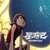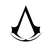# 2018 程序设计能力实训第一次机考分析（部分）

ultmaster edited 2 年，5 月前

### 上午

#### E.

LL dfs(int x, int af, int bf, int kf) {
if (x < 0) return 1;
LL ret = 0;
for (int i = 0; i <= (af ? 1 : a[x]); ++i)
for (int j = 0; j <= (bf ? 1 : b[x]); ++j) {
if (kf || (i & j) <= k[x])
ret += dfs(x - 1, af || i < a[x], bf || j < b[x],
kf || ((i & j) < k[x]));
}
return ret;
}


### 下午

#### E.